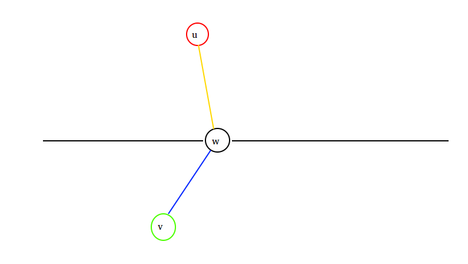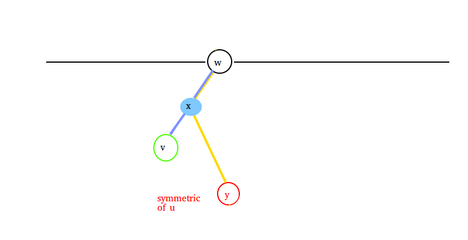# Meena Raju and Two Treesovis96Criterion 2021 Round 9

This is an easy problem.

There are two cases we need to solve, and they are,

1. The two nodes belong to same tree or
2. They belong to different trees

For the first case, solution is simply the shortest path in the initial tree, because of merging two similar trees it can be proven that there is no shorter solution. The actual problem is in the second case.

So, If the two nodes are from two different trees then one thing is sure that their shortest path contains one of the deaf nodes of the tree. Let, $dist(u, v)$ be the shortest path between node $u$ and $v$. So for every deaf node $d_i$ we need to find minimum of $dist(u, d_i) + dist(d_i, v)$. Suppose you have found the shortest path and optimal deaf node is $w$. Let's say $u$ is from the upper tree and $v$ is from the lower tree. Then we can picture our imagination like this,Now because of these two trees are same (also symmetric) we can move $u$ to the lower tree. Suppose symmetric node of u is $y$ and if we picture that one, it will also look like,Here you can see another node $x$. By this node x these two paths go into to separate ways. Also, $y - x - v$ is a unique path in the lower tree which is also exactly $dist(y, v)$, and the path between $x$ and $w$ is counted twice. From this little observation we can say that, the solution for the nodes which belongs to two different trees is,

$dist(u, v) = dist(y, v) + 2 * (minimum\ distance\ between\ the\ deaf\ nodes\ and\ the\ path\ between\ y\ and\ v)$

To solve this problem one can pre-calculate the minimum distance from the deaf nodes to all the other nodes. And then path minimum and distance queries. Which one can easily do with the lca and sparse table.

### Statistics

33% Solution Ratio
shefinEarliest, Feb '21
shefinFastest, 0.9s
shefinLightest, 27 MB
shefinShortest, 3706B